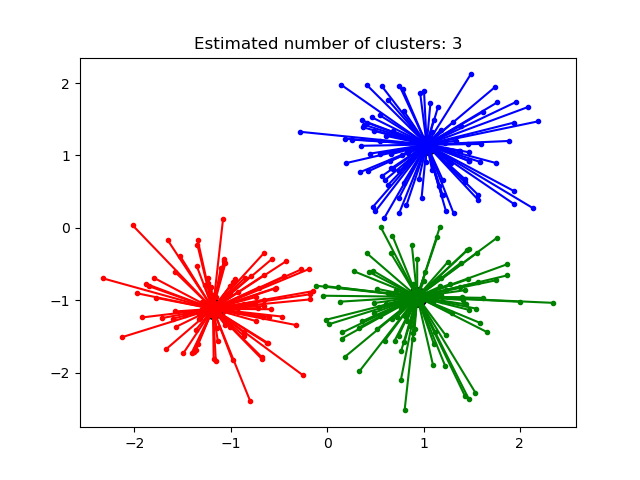# Demo of affinity propagation clustering algorithm¶

Reference: Brendan J. Frey and Delbert Dueck, “Clustering by Passing Messages Between Data Points”, Science Feb. 2007Out:

/home/circleci/project/sklearn/cluster/_affinity_propagation.py:146: FutureWarning: 'random_state' has been introduced in 0.23. It will be set to None starting from 0.25 which means that results will differ at every function call. Set 'random_state' to None to silence this warning, or to 0 to keep the behavior of versions <0.23.
warnings.warn(("'random_state' has been introduced in 0.23. "
Estimated number of clusters: 3
Homogeneity: 0.872
Completeness: 0.872
V-measure: 0.872
Silhouette Coefficient: 0.753


print(__doc__)

from sklearn.cluster import AffinityPropagation
from sklearn import metrics
from sklearn.datasets import make_blobs

# #############################################################################
# Generate sample data
centers = [[1, 1], [-1, -1], [1, -1]]
X, labels_true = make_blobs(n_samples=300, centers=centers, cluster_std=0.5,
random_state=0)

# #############################################################################
# Compute Affinity Propagation
af = AffinityPropagation(preference=-50).fit(X)
cluster_centers_indices = af.cluster_centers_indices_
labels = af.labels_

n_clusters_ = len(cluster_centers_indices)

print('Estimated number of clusters: %d' % n_clusters_)
print("Homogeneity: %0.3f" % metrics.homogeneity_score(labels_true, labels))
print("Completeness: %0.3f" % metrics.completeness_score(labels_true, labels))
print("V-measure: %0.3f" % metrics.v_measure_score(labels_true, labels))
print("Silhouette Coefficient: %0.3f"
% metrics.silhouette_score(X, labels, metric='sqeuclidean'))

# #############################################################################
# Plot result
import matplotlib.pyplot as plt
from itertools import cycle

plt.close('all')
plt.figure(1)
plt.clf()

colors = cycle('bgrcmykbgrcmykbgrcmykbgrcmyk')
for k, col in zip(range(n_clusters_), colors):
class_members = labels == k
cluster_center = X[cluster_centers_indices[k]]
plt.plot(X[class_members, 0], X[class_members, 1], col + '.')
plt.plot(cluster_center, cluster_center, 'o', markerfacecolor=col,
markeredgecolor='k', markersize=14)
for x in X[class_members]:
plt.plot([cluster_center, x], [cluster_center, x], col)

plt.title('Estimated number of clusters: %d' % n_clusters_)
plt.show()


Total running time of the script: ( 0 minutes 0.328 seconds)

Gallery generated by Sphinx-Gallery